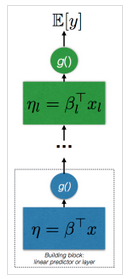# 广义线性模型（GLMs）

$$\eta=\beta^Tx+\beta_0$$

$$y = \eta+\epsilon \qquad \epsilon \sim \mathcal{N}(0,\sigma^2)$$

在此公式中， $\eta$是该模型的系统成分， $\eta$是随机扰动项。广义线性模型（GLMs） 使我们能够对这一模型进行扩展，允许因变量的分布不局限于高斯分布而扩展到更广泛的分布（例如典型的指数分布族）。在这种情况下，我们可以写出广义回归问题，结合系数和偏置为更紧凑的表示法，如：

$$\eta = \beta^\top x, \qquad \beta=[\hat \beta, \beta_0], x = [\hat{x}, 1]$$

$$\mathbb{E}[y] = \mu = g^{-1}(\eta)$$

# 递归广义线性模型

$$h_l(x) = f_l(\eta_l)$$$$\mathbb{E}[y] = \mu_L = h_L \circ \ldots \circ h_1 \circ h_o(x)$$

# 学习和估计

$$\mathcal{L} = – \log p(y | \mu_L)$$

# 参考文献

 Christopher M Bishop, Neural networks for pattern recognition, 1995

 Peter McCullagh, John A Nelder, Generalized linear models., 1989

 Peter J Bickel, Kjell A Doksum, Mathematical Statistics, volume I, 2001

 Leon Bottou, Stochastic Gradient Descent Tricks, Neural Networks: Tricks of the Trade, 2012